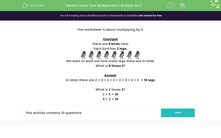# Know Your Multiplication: Multiply by 2

In this worksheet, students will learn to multiply by 2.Key stage:  KS 1

Curriculum topic:   Number: Multiplication and Division

Curriculum subtopic:   Use Multiplication/Division Facts (2, 5, 10)

Popular topics:   Multiplication worksheets

Difficulty level:#### Worksheet Overview

This activity is about multiplying by 2.

Example

There are 8 birds here.

Each bird has 2 legs.We want to work out how many legs there are in total.

What is 8 lots of 2?

In total, there are 2 + 2 + 2 + 2 + 2 + 2 + 2 + 2  = 16 legs

What is 8 times 2?

8 x 2 = 16

2 x 8 = 16

Let's look at another example.There are 6 cats above and each of them has 2 eyes.

How many eyes are there altogether?

What is 6 lots of 2?

In total, there are 2 + 2 + 2 + 2  + 2 + 2 = 12 eyes.

There are 12 eyes.

6 x 2 = 12

2 x 6 = 12

How do you feel about tackling some questions now?### What is EdPlace?

We're your National Curriculum aligned online education content provider helping each child succeed in English, maths and science from year 1 to GCSE. With an EdPlace account you’ll be able to track and measure progress, helping each child achieve their best. We build confidence and attainment by personalising each child’s learning at a level that suits them.

Get started